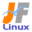Linux Kernel 2.6 Documentation: /usr/src/linux/Documentation/device-mapper/snapshot.txt

device-mapper/snapshot.txt

Linux 2.6 \$B\$N%G%P%\$%9%^%C%Q!<\$K\$h\$k(B LVM \$B\$N%9%J%C%W%7%g%C%H5!G=\$N2r@b(B [\$B%W%l%\$%s%F%-%9%HHG(B]
• \$B86Cx:n
• \$BK]Lu
• \$B%P!<%8%g%s(B: 2.6.16
• \$BK]LuF|;~(B: 2006/07/18
```

Device-mapper snapshot support
==============================

\$B%G%P%\$%9%^%C%Q!<(B \$B%9%J%C%W%7%g%C%H%5%]!<%H(B
=========================================

Device-mapper allows you, without massive data copying:

\$B%G%P%\$%9%^%C%Q!<\$O5pBg\$J%G!<%?%3%T!<\$J\$7\$G2<5-\$r2DG=\$K\$7\$^\$9!'(B

*) To create snapshots of any block device i.e. mountable, saved states of
the block device which are also writable without interfering with the
original content;

*) \$B\$"\$i\$f\$k%V%m%C%/%G%P%\$%9\$N%9%J%C%W%7%g%C%H(B (\$B!a%^%&%s%H2DG=\$G!"85\$N(B
\$BFbMF\$K1F6A\$rM?\$(\$J\$\$=q\$-9~\$_\$b2DG=\$J%V%m%C%/%G%P%\$%9\$NJ]B8\$5\$l\$?>uBV(B)
\$B\$N:n@.(B

*) To create device "forks", i.e. multiple different versions of the
same data stream.

*) \$B%G%P%\$%9\$N!VJ,4t!W(B(\$B!aF1\$8%G!<%?%9%H%j!<%`\$NJ#?t\$N0[\$J\$k%P!<%8%g%s(B)
\$B\$N:n@.(B

In both cases, dm copies only the chunks of data that get changed and
uses a separate copy-on-write (COW) block device for storage.

\$B\$I\$A\$i\$N>l9g\$G\$b!"%G%P%\$%9%^%C%Q!<\$OJQ99\$5\$l\$?%G!<%?\$N2t!J(Bchunk\$B!'LuCm(B
1) \$B\$H%9%H%l!<%8\$KJ,N%\$5\$l\$?(B copy-on-write (COW\$B!'LuCm(B 2) \$B%V%m%C%/%G%P%\$(B
\$B%9\$N\$_%3%T!<\$7\$^\$9!#(B

\$BLuCm(B 1: \$B%V%m%C%/%G%P%\$%9\$K\$*\$1\$k%V%m%C%/\$NC10L=89g?t\$r<(\$9MQ8l!#%G%#%9(B
\$B%/%"%/%;%9;~\$J\$I!"\$"\$kDxEY\$^\$H\$^\$C\$??t\$N%V%m%C%/(B I/O \$B\$,\$"\$C\$?J}\$,@-G=(B
\$B\$,=P\$?\$j4IM}NN0h\$,@aLs\$G\$-\$?\$j\$9\$k\$,!"\$=\$N:]\$NC10L%V%m%C%/?t\$r;X\$9!#(B
\$B%U%!%\$%k%7%9%F%`\$d%=%U%H%&%'%"(B RAID \$B\$N%I%-%e%a%s%H;2>H!#(B

\$BLuCm(B 2: copy-on-write (COW) \$B!D=q\$-9~\$_\$K\$h\$k%G!<%?99?7\$,\$"\$C\$?ItJ,\$@\$1(B
\$B?7\$?\$KNN0h\$r3NJ]\$7\$F\$=\$3\$K99?7%G!<%?\$r=q\$-9~\$`J}<0\$N;v!#99?7\$N\$J\$\$ItJ,(B
\$B\$O85\$NNN0h\$r;HMQ\$9\$k0Y!"L5BL\$,>/\$J\$\$!#(BLinux \$B\$N%a%b%j4IM}\$d3FA[%^%7(B
\$B%s\$N%G%#%9%/%9%J%C%W%7%g%C%HEy\$N%I%-%e%a%s%H;2>H!#(B

There are two dm targets available: snapshot and snapshot-origin.
\$B#2\$D\$N%G%P%\$%9%^%C%Q!<%?!<%2%C%H(B(snapshot, snapshot-origin)\$B\$,MxMQ2DG=(B
\$B\$G\$9!#(B

*) snapshot-origin <origin>

which will normally have one or more snapshots based on it.
Reads will be mapped directly to the backing device. For each write, the
original data will be saved in the <COW device> of each snapshot to keep
its visible content unchanged, at least until the <COW device> fills up.

\$B\$3\$l\$ODL>o%*%j%8%J%k\$K#1\$D\$^\$?\$O\$=\$l0J>e\$N%9%J%C%W%7%g%C%H\$,\$"\$j\$^\$9!#(B
\$BFI\$_9~\$_\$OD>@\%P%C%/%"%C%W%G%P%\$%9\$K%^%C%T%s%0\$5\$l\$^\$9!#3F=q\$-9~\$_\$G\$O!"(B
\$B8+\$?L\\$NFbMF\$KJQ99\$J\$\$\$h\$&\$K!">/\$J\$/\$H\$b(B <COW \$B%G%P%\$%9(B> \$B\$,\$\$\$C\$Q\$\$\$K\$J(B
\$B\$k\$^\$G%*%j%8%J%k%G!<%?\$r3F%9%J%C%W%7%g%C%H\$N(B <COW \$B%G%P%\$%9(B> \$B\$KJ]B8\$7\$^(B
\$B\$9!#(B

*) snapshot <origin> <COW device> <persistent?> <chunksize>

A snapshot of the <origin> block device is created. Changed chunks of
<chunksize> sectors will be stored on the <COW device>.  Writes will
only go to the <COW device>.  Reads will come from the <COW device> or
from <origin> for unchanged data.  <COW device> will often be
smaller than the origin and if it fills up the snapshot will become
useless and be disabled, returning errors.  So it is important to monitor
the amount of free space and expand the <COW device> before it fills up.

<\$B85\$N(B>\$B%V%m%C%/%G%P%\$%9\$N%9%J%C%W%7%g%C%H\$,:n\$i\$l\$^\$9!#(B<chunksize>\$B%;%/(B
\$B%?(B (\$BLuCm(B3) \$BJQ99\$5\$l\$?%A%c%s%/\$,(B <COW \$B%G%P%\$%9(B> \$B>e\$KJ]B8\$5\$l\$^\$9!#=q\$-(B
\$B9~\$_\$OC1\$K(B <COW \$B%G%P%\$%9(B> \$B>e\$K9T\$-\$^\$9!#FI\$_9~\$_\$O(B <COW \$B%G%P%\$%9(B> \$B\$^\$?(B
\$B\$OL5JQ99%G!<%?\$O(B <origin> \$B\$+\$i9T\$o\$l\$^\$9!#(B<COW \$B%G%P%\$%9(B> \$B\$O\$7\$P\$7\$P(B
origin \$B\$h\$j>.\$5\$/!"\$3\$l\$,\$\$\$C\$Q\$\$\$K\$J\$C\$?\$i(B snapshot \$B\$O;H\$\$\$b\$N\$K\$J\$i(B
\$B\$:!"L58z2=\$5\$l\$F\$7\$^\$\$!"%(%i!<\$,JV\$5\$l\$^\$9!#\$J\$N\$G!"6u\$-%9%Z!<%9\$NNL\$N(B
\$B4F;k\$H!"\$\$\$C\$Q\$\$\$K\$J\$kA0\$N(B <COW \$B%G%P%\$%9(B> \$B\$N3HD%\$O=EMW\$G\$9!#(B

\$BLuCm(B3\$B!'(B1\$B%;%/%?!a\$3\$3\$G\$O(B 512 \$B%P%\$%H(B

<persistent?> is P (Persistent) or N (Not persistent - will not survive
after reboot).

<persistent?> \$B\$O(B P (Persistent\$B!a1JB3(B) \$B\$^\$?\$O(B N (Not persistent\$B!aHs1JB3(B
\$B!a:F5/F08e\$O;D\$i\$J\$\$(B) \$B\$G\$9!#(B

The difference is that for transient snapshots less metadata must be
saved on disk - they can be kept in memory by the kernel.

\$B\$3\$l\$i\$N0c\$\$\$O!"0l;~E*%9%J%C%W%7%g%C%H\$N0Y\$K\$O%G%#%9%/\$KJ]B8\$5\$l\$k%a%?(B
\$B%G!<%?\$,\$h\$j>.\$5\$\$!a\$3\$l\$i\$O%+!<%M%k\$K\$h\$C\$F%a%b%j>e\$KJ]B8\$5\$l\$k\$H\$\$\$&(B
\$B;v\$G\$9!#(B

How this is used by LVM2
========================

LVM2 \$B\$G\$N%9%J%C%W%7%g%C%H\$N;H\$\$J}(B
=================================

When you create the first LVM2 snapshot of a volume, four dm devices are used:

\$B:G=i\$K\$"\$k%\%j%e!<%`\$N(B LVM2 \$B%9%J%C%W%7%g%C%H\$r:n@.\$9\$k:]!"#4\$D\$N%G%P%\$(B
\$B%9%^%C%Q!<%G%P%\$%9\$,;HMQ\$5\$l\$^\$9!#(B

1) a device containing the original mapping table of the source volume;

1) \$B%=!<%9%\%j%e!<%`\$N85!9\$N%^%C%T%s%0%F!<%V%k\$r;}\$D%G%P%\$%9(B

2) a device used as the <COW device>;

2) <COW \$B%G%P%\$%9(B> \$B\$H\$7\$F;H\$o\$l\$k%G%P%\$%9(B

3) a "snapshot" device, combining #1 and #2, which is the visible snapshot
volume;

3) \$B2D;k%9%J%C%W%7%g%C%H%\%j%e!<%`\$H\$7\$F\$N!V%9%J%C%W%7%g%C%H!W%G%P%\$%9(B
(1 \$B\$H(B 2 \$B\$NAH\$_9g\$o\$;(B)

4) the "original" volume (which uses the device number used by the original
source volume), whose table is replaced by a "snapshot-origin" mapping
from device #1.

4) 1\$BHVL\\$N%G%P%\$%9\$+\$i!V(Bsnapshot-origin\$B!W%^%C%T%s%0\$K%F!<%V%k\$,CV49\$5(B
\$B\$l\$?!V85!9\$N%\%j%e!<%`!W(B(\$B85!9\$N%=!<%9%\%j%e!<%`\$,;H\$C\$F\$\$\$?%G%P%\$%9(B
\$BHV9f\$r;HMQ\$9\$k(B)

A fixed naming scheme is used, so with the following commands:

\$B8GDjL?L>5,B'\$,;HMQ\$5\$l\$k\$N\$G!"uBV\$K\$J\$j\$^\$9(B (\$B%\%j%e!<%`\$,2<5-\$N=gHV(B)\$B!'(B

# dmsetup table|grep volumeGroup
volumeGroup-base-real: 0 2097152 linear 8:19 384
volumeGroup-snap-cow: 0 204800 linear 8:19 2097536
volumeGroup-snap: 0 2097152 snapshot 254:11 254:12 P 16
volumeGroup-base: 0 2097152 snapshot-origin 254:11

# ls -lL /dev/mapper/volumeGroup-*
brw-------  1 root root 254, 11 29 ago 18:15 /dev/mapper/volumeGroup-base-real
brw-------  1 root root 254, 12 29 ago 18:15 /dev/mapper/volumeGroup-snap-cow
brw-------  1 root root 254, 13 29 ago 18:15 /dev/mapper/volumeGroup-snap
brw-------  1 root root 254, 10 29 ago 18:14 /dev/mapper/volumeGroup-base

```